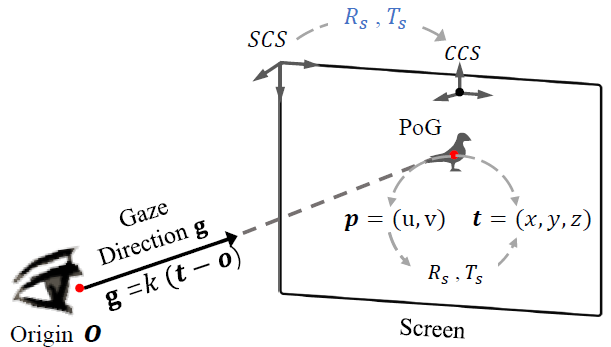# 2D/3D Gaze Conversion

3D gaze means gaze directions originating from subjects and pointing to gaze targets.
2D gaze means the point of gaze (PoG). It is also the coordinate of gaze targets in the screen. We illustrate them in the figure below.``````@article{Cheng2021Survey,
title={Appearance-based Gaze Estimation With Deep Learning: A Review and Benchmark},
author={Yihua Cheng and Haofei Wang and Yiwei Bao and Feng Lu},
journal={arXiv preprint arXiv:2104.12668},
year={2021}
}
``````

## Tutorial

### 3D Gaze To 2D Gaze

We provide an example.

``````gazepoint =  Gaze3DTo2D(gazedirection, gazeorigin, rmat, tmat, require3d)
``````

We also provide specific conversion codes in each dataset.

To use the specific codes, you first should download the core code and rename the code as `"conversion.py"`. The document about the specific codes is coming soon.

### 2D Gaze To 3D Gaze

We also provide an example.

``````gazepoint = Gaze2DTo3D(point, origin, rmat, tmat)
``````

We also provide specific conversion codes in each dataset.

To use the specific codes, you first should download the core code and rename the code as `"conversion.py"`. The document about the specific codes is coming soon.

## Document

``````Gaze2DTo3D(point, origin, rmat, tmat)
``````

Paramters:

point (narray) : the coordinate of PoR in the screen. The shape is (2, ).

origin (narray) : The origin of gaze direction in CCS. The shape is (3, ).

rmat (narray) : The rotation matrix. It is used to convert points in SCS to CCS. The shape is (3, 3).

rmat (narray) : The translation vector. It is used to convert points in SCS to CCS. The shape is (3, 1).

Return gaze

gaze (narray) : Gaze direction. The gaze direction originates from the `origin`. The shape is (3,)

``````Gaze3DTo2D(gaze, origin, rmat, tmat, require3d=False)
``````

Paramters:

gaze (narray) : gaze direction. The shape is (3, ).

origin (narray) : The origin of gaze direction in CCS. The shape is (3, ).

rmat (narray) : The rotation matrix. It is used to convert points in SCS to CCS. The shape is (3, 3).

rmat (narray) : The translation vector. It is used to convert points in SCS to CCS. The shape is (3, 1).

require3d (bool) : Decide whether to keep the value in the z-dimesion.

Return gaze

gaze (narray) : If `require3d` is True, return the 3D vector of PoR, where the z-axis is usually zero. If `require3d` is False, return the PoR.